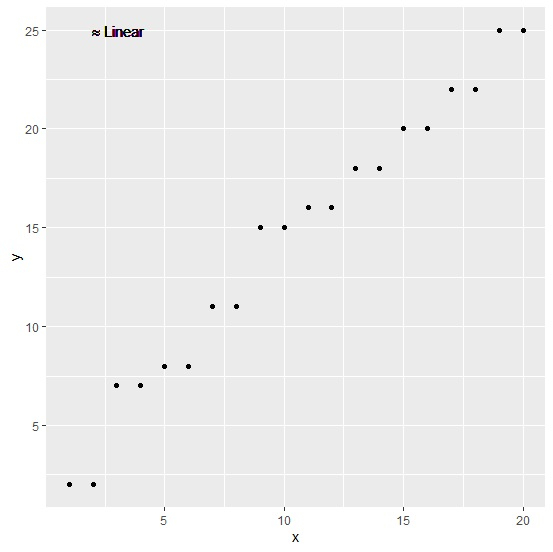R ProgrammingServer Side ProgrammingProgramming

To add approximately equal sign in a plot using ggplot2, we can use tilde sign twice as ~~ in geom_text function of ggplot2 package. For example, we can do this by using the following syntax geom_text(aes(label="NULL%~~%")). Check out the below example to understand how it works.

## Example

Consider the below data frame −

Live Demo

x<-1:20
y<-rep(c(2,7,8,11,15,16,18,20,22,25),each=2)
df<-data.frame(x,y)
df

## Output

    x   y
1   1   2
2   2   2
3   3   7
4   4   7
5   5   8
6   6   8
7   7  11
8   8  11
9   9  15
10 10  15
11 11  16
12 12  16
13 13  18
14 14  18
15 15  20
16 16  20
17 17  22
18 18  22
19 19  25
20 20  25

Loading ggplot2 package and creating a scatterplot between x and y with some text showing approximately equal inside the plot −

## Example

library(ggplot2)
ggplot(df,aes(x,y))+geom_point()+geom_text(aes(label="NULL%~~%Linear",x=3,y=25),parse=T)

## Output